# RD Sharma Solutions for Class 10 Maths Chapter 8 Quadratic Equations Exercise 8.6

A quadratic equation can have at most two roots. But whether a quadratic equation can have 1, 2 or even no roots is decided by finding the nature of roots. RD Sharma Solutions Class 10 exercise 8.6 contains problems based explicitly on the nature of roots. Detailed solutions developed by experts can be accessed in the RD Sharma Solutions for Class 10 Maths Chapter 8 Quadratic Equations Exercise 8.6 PDF provided below.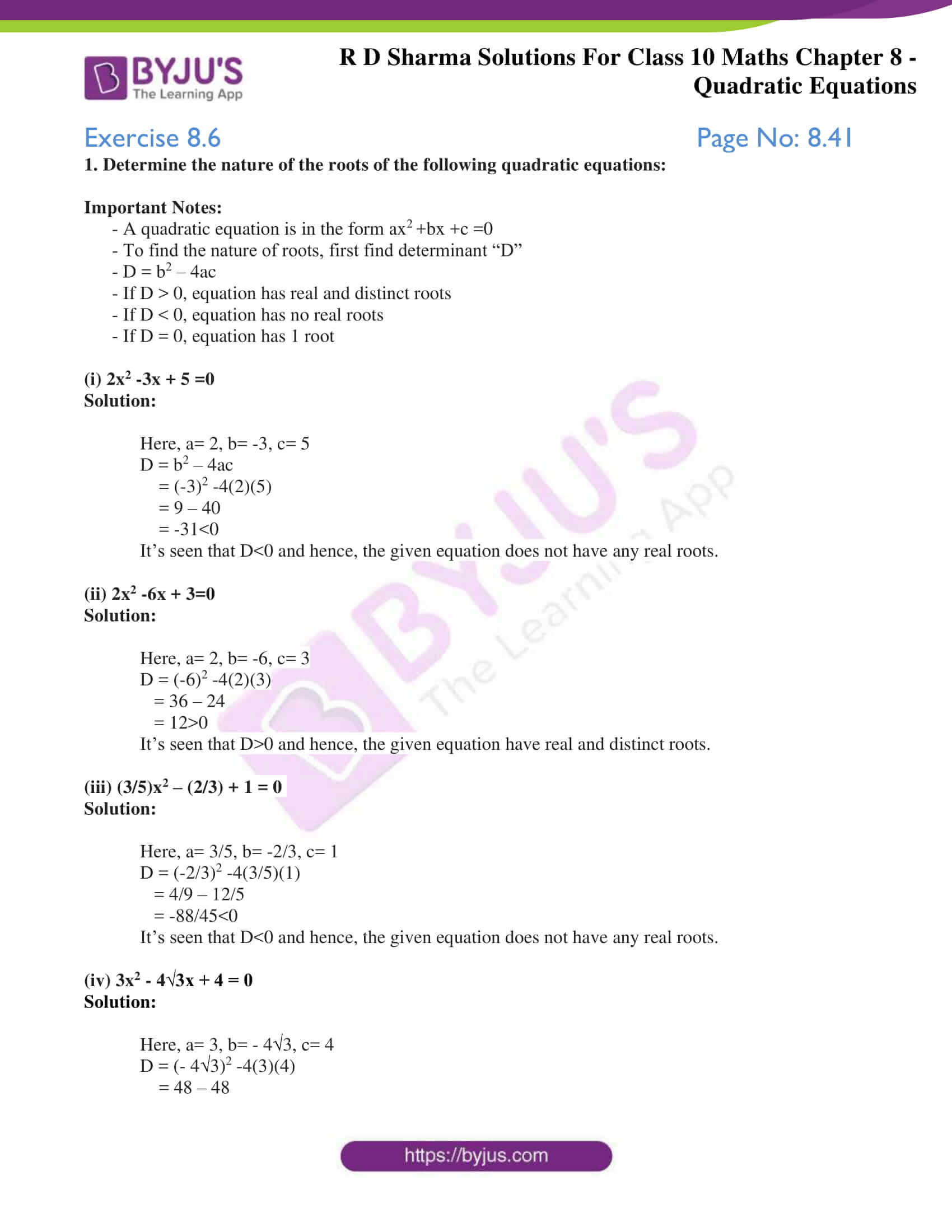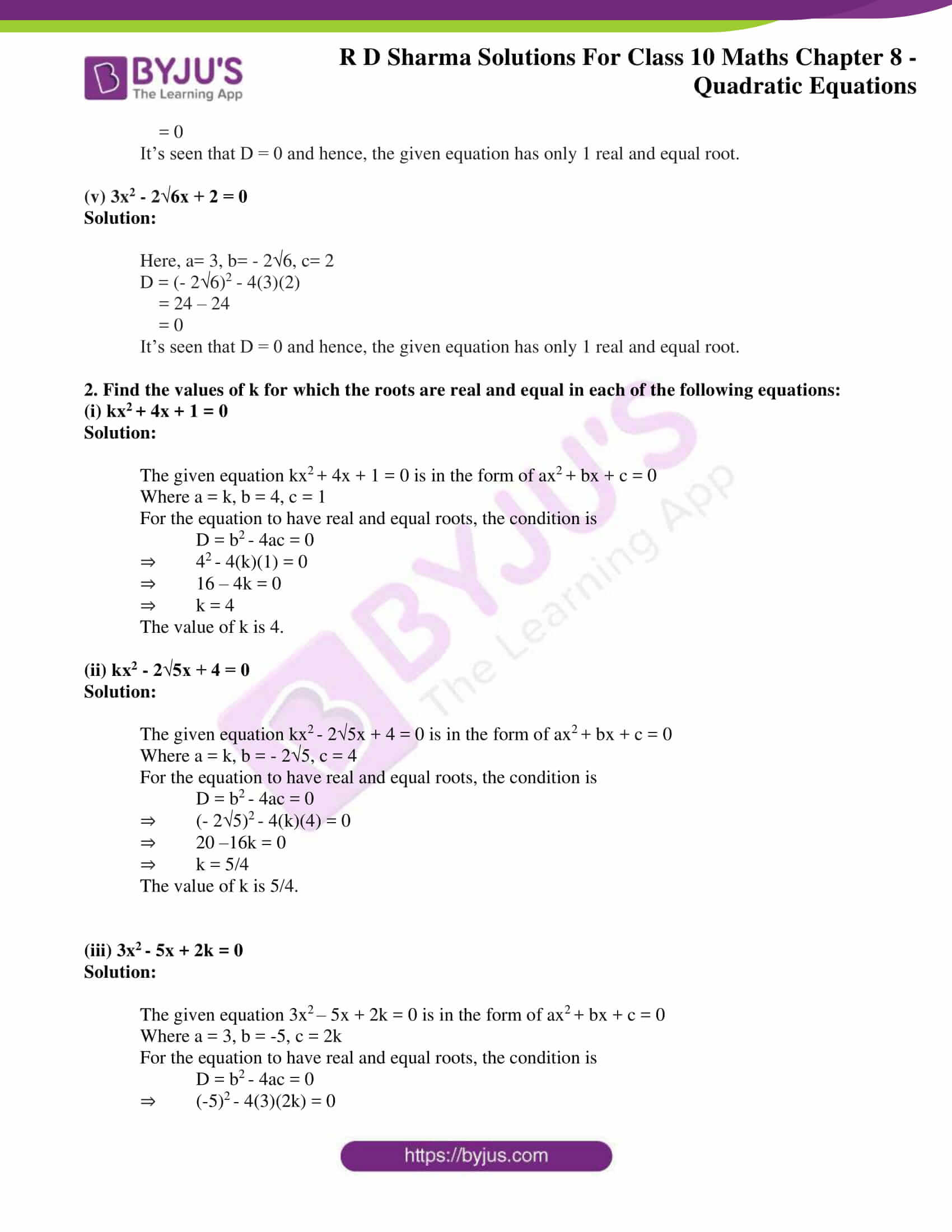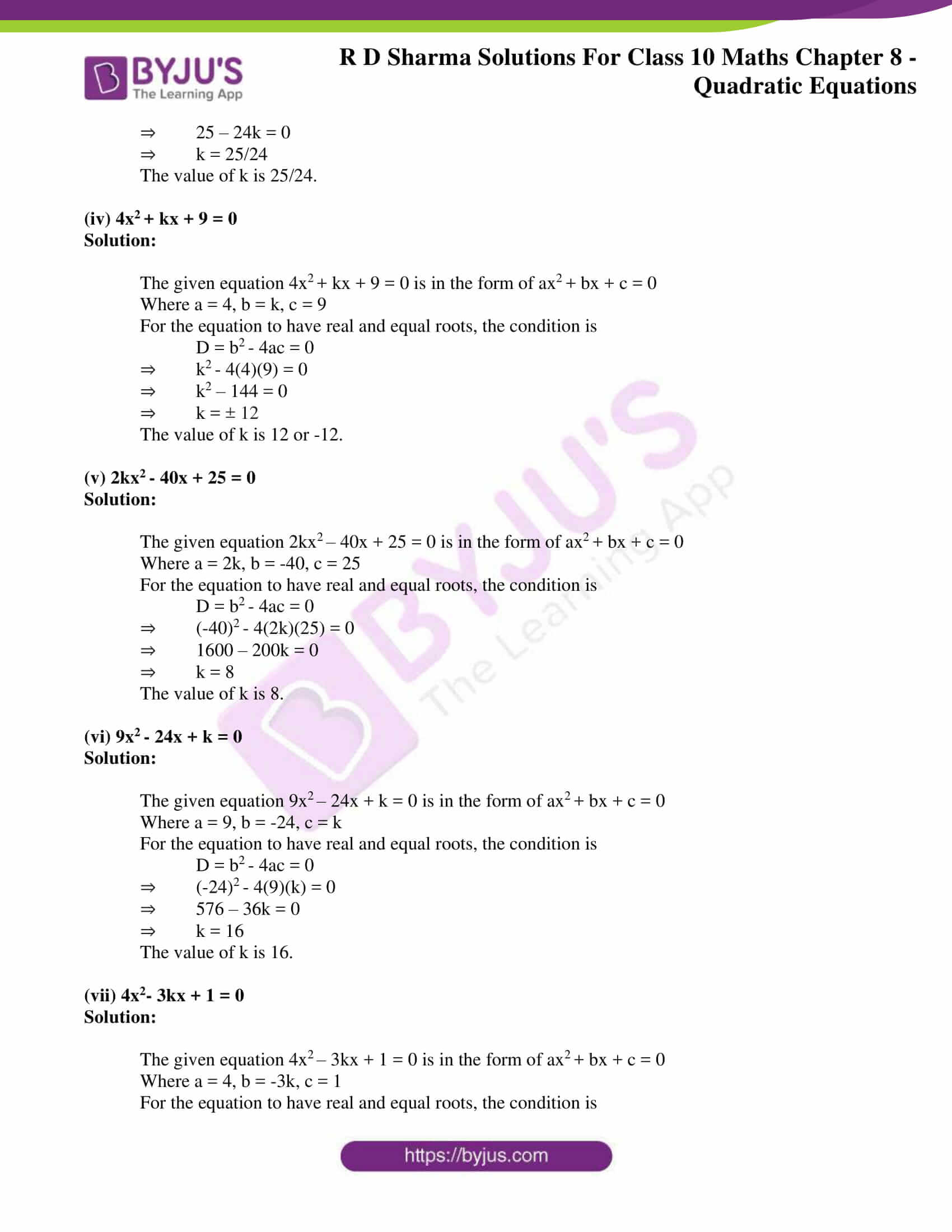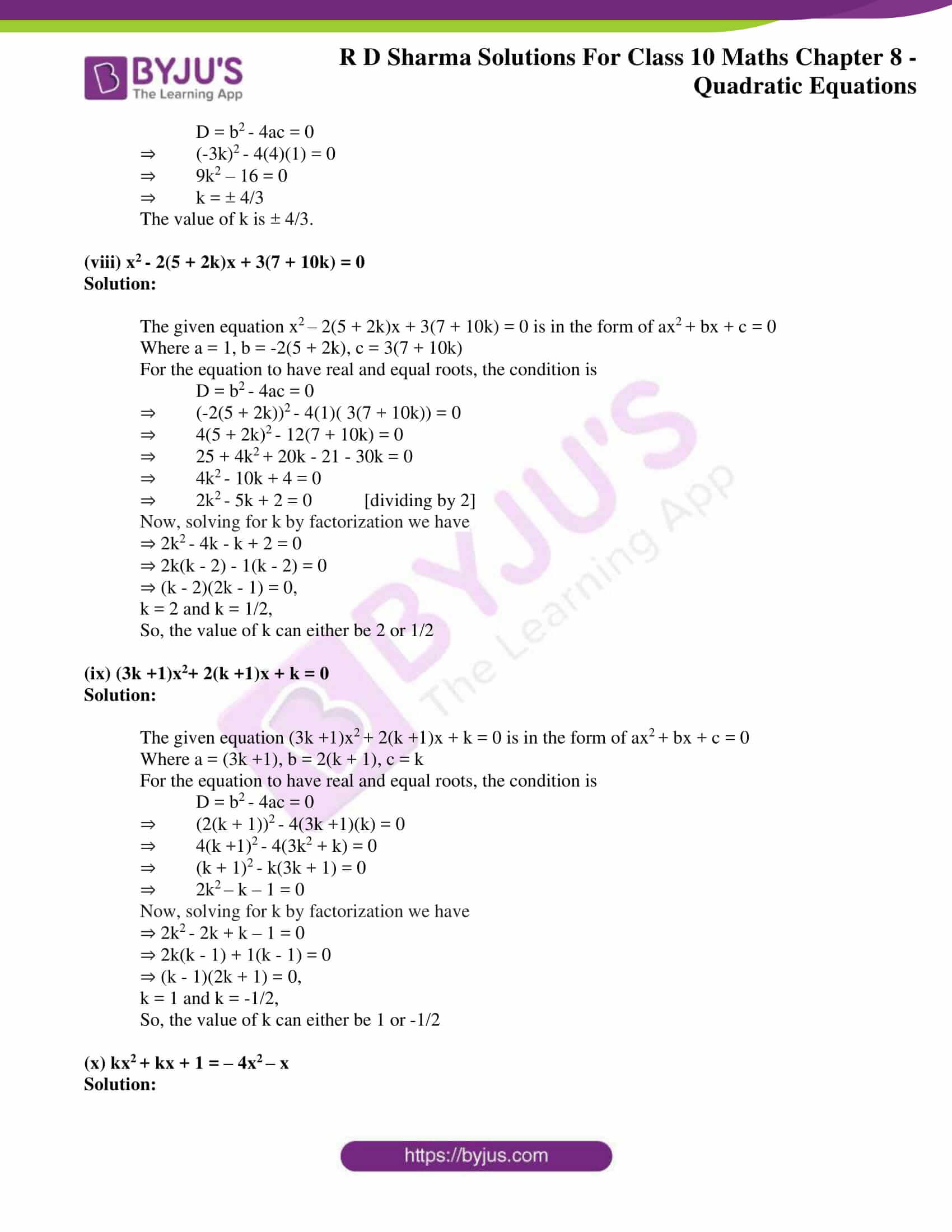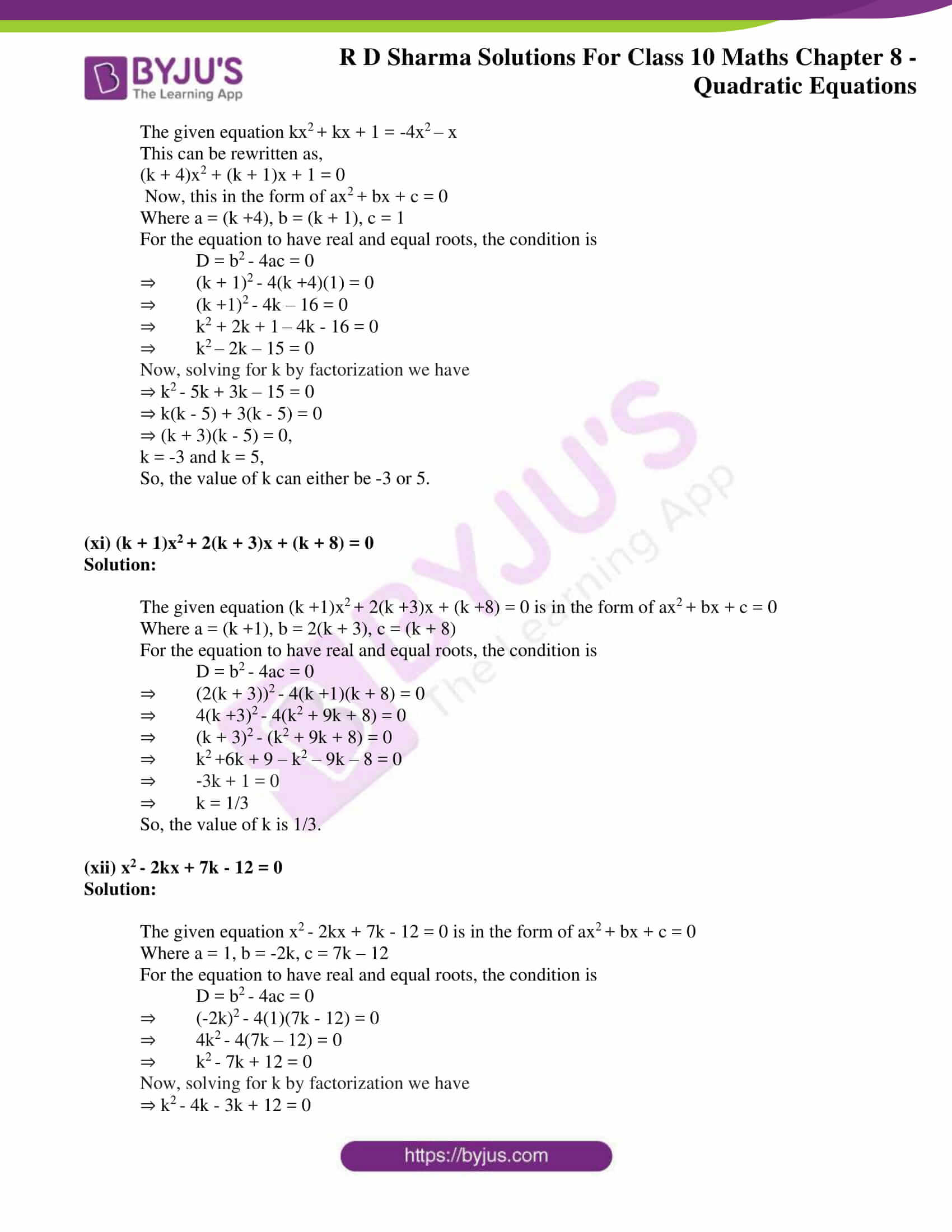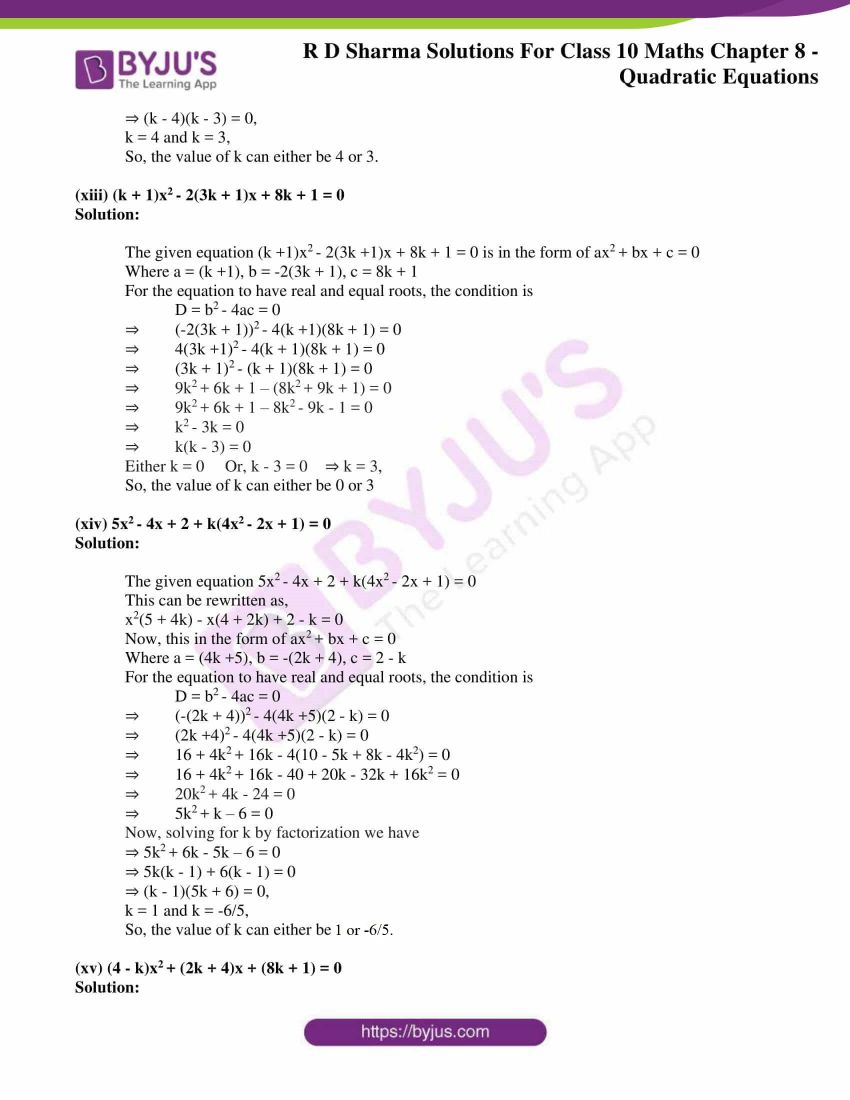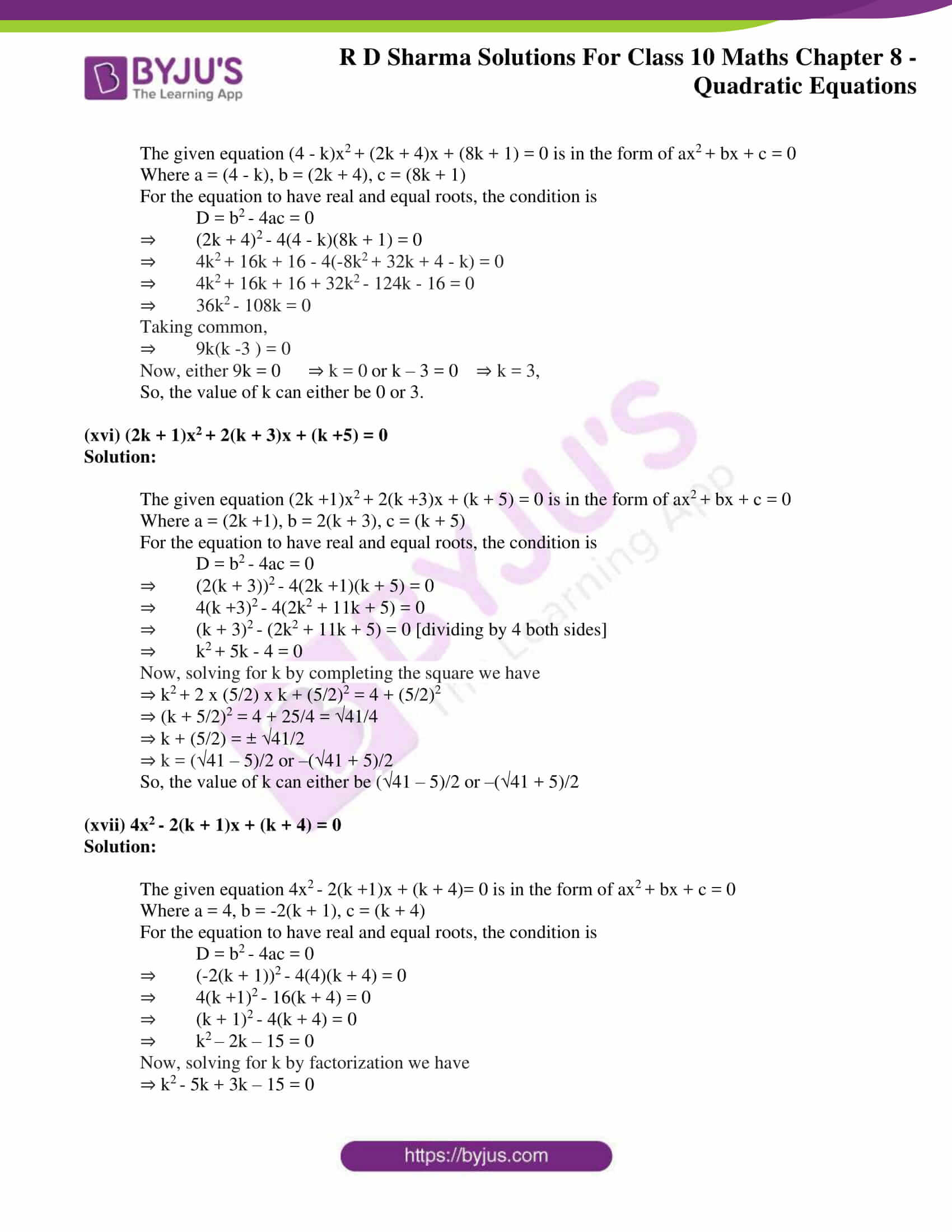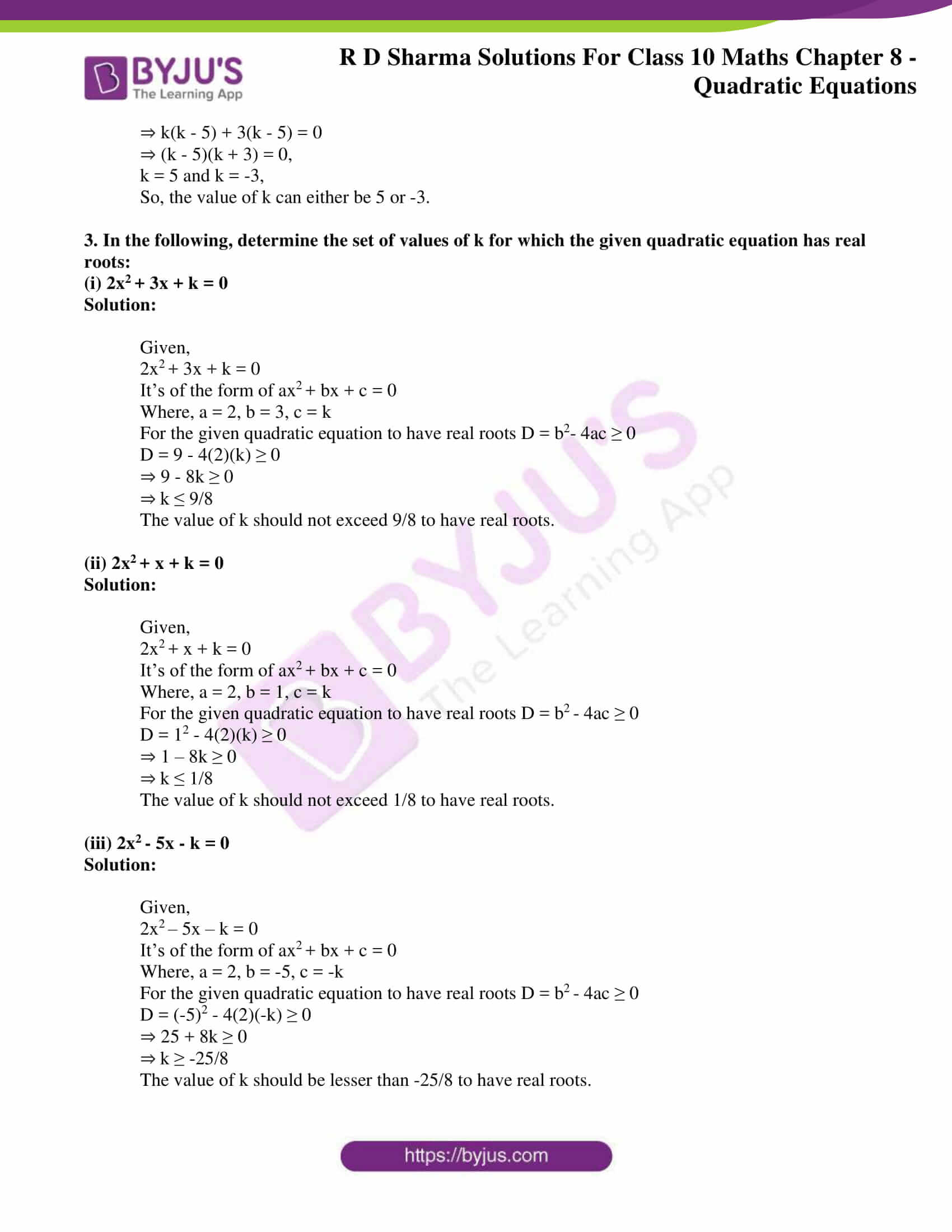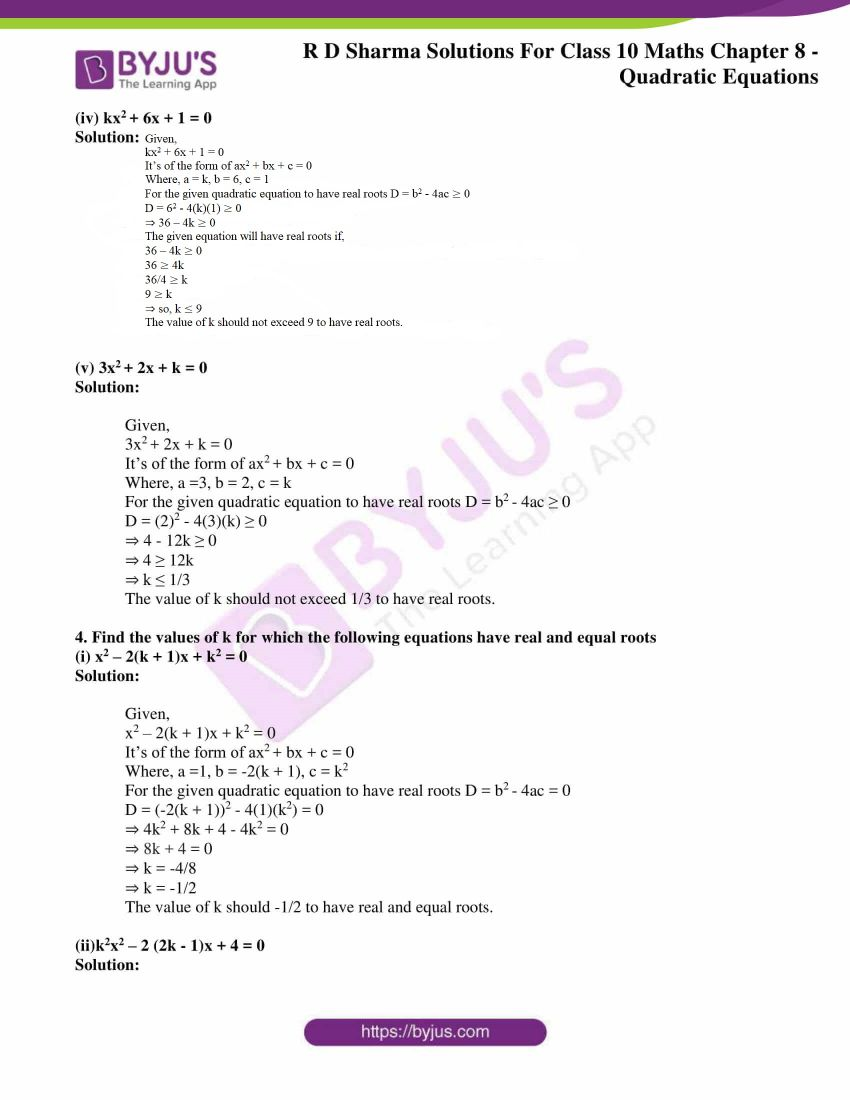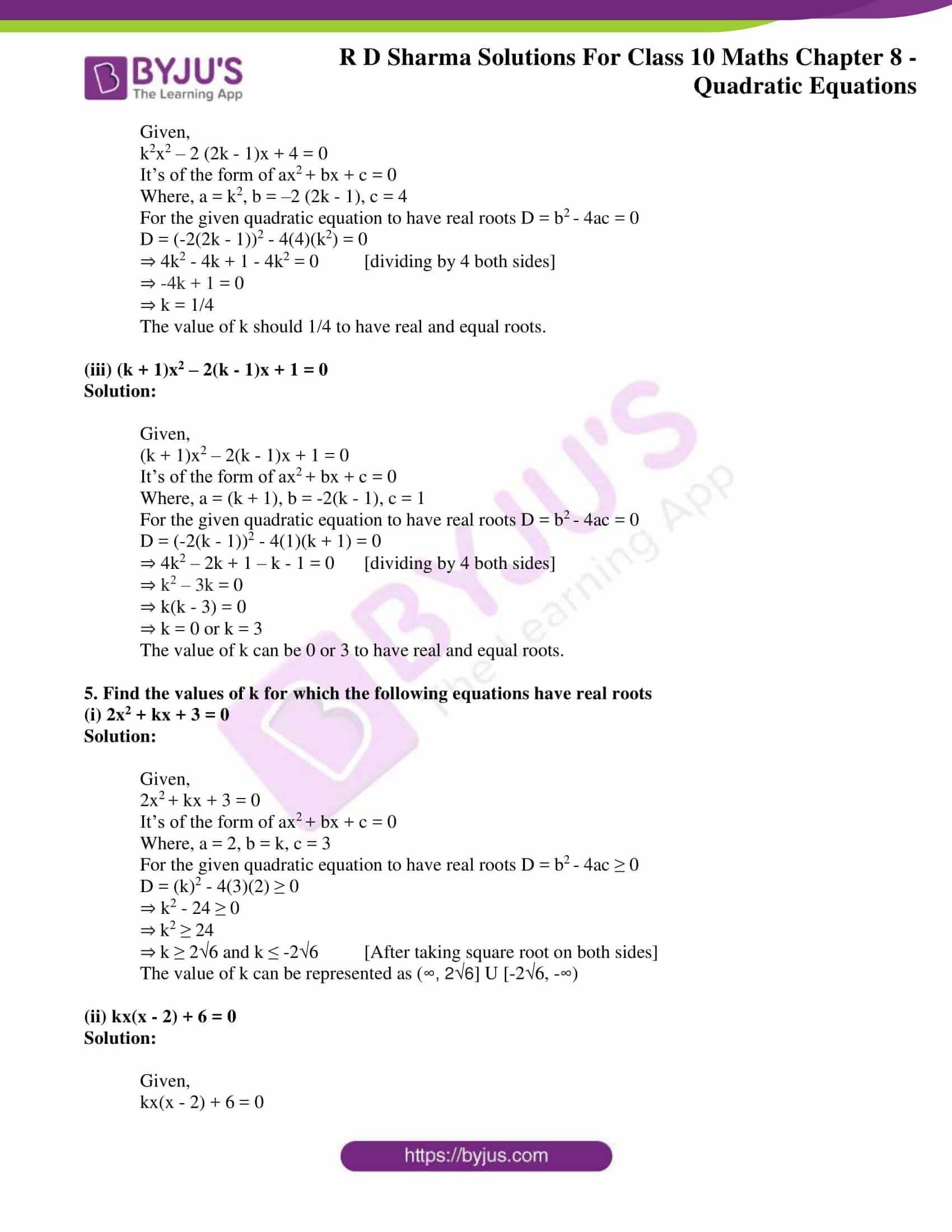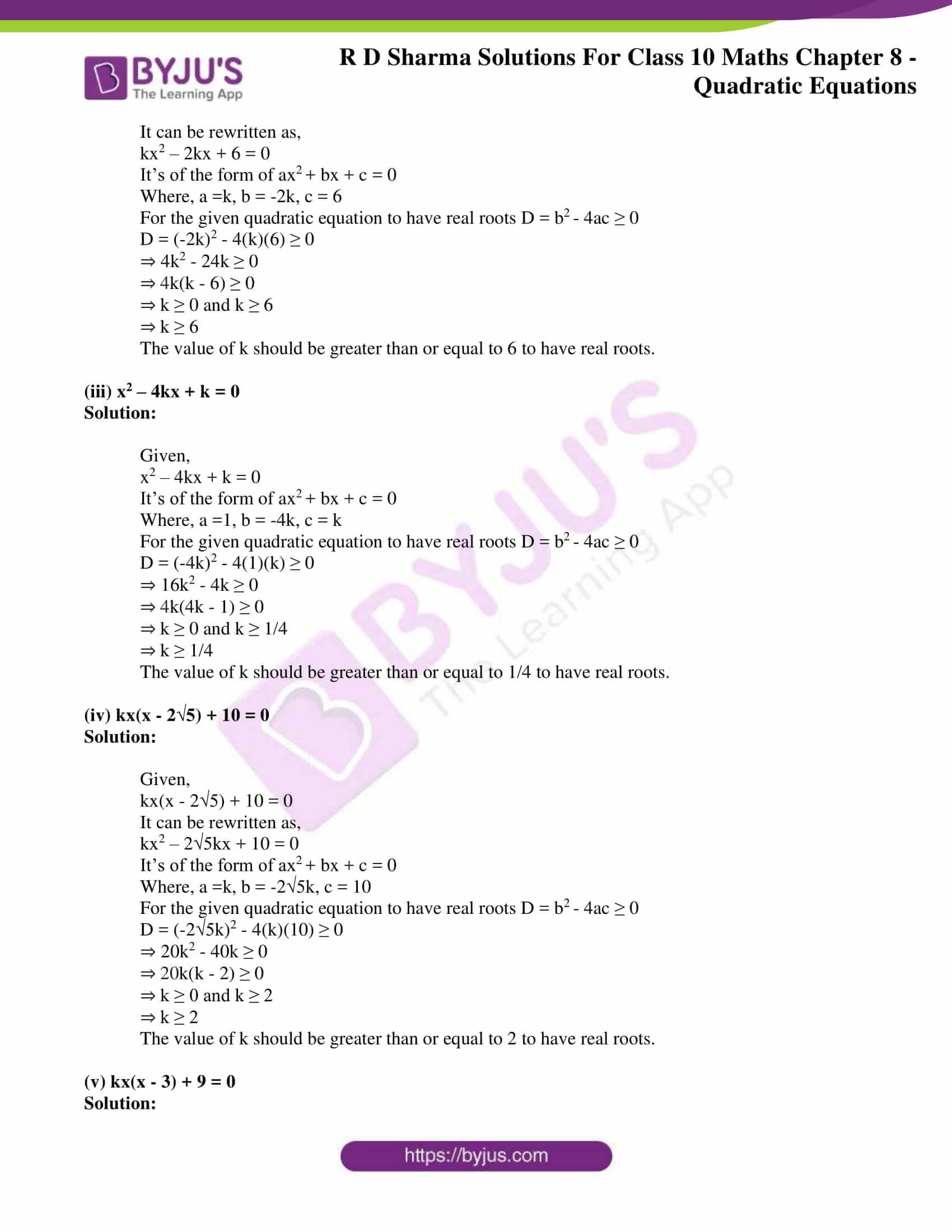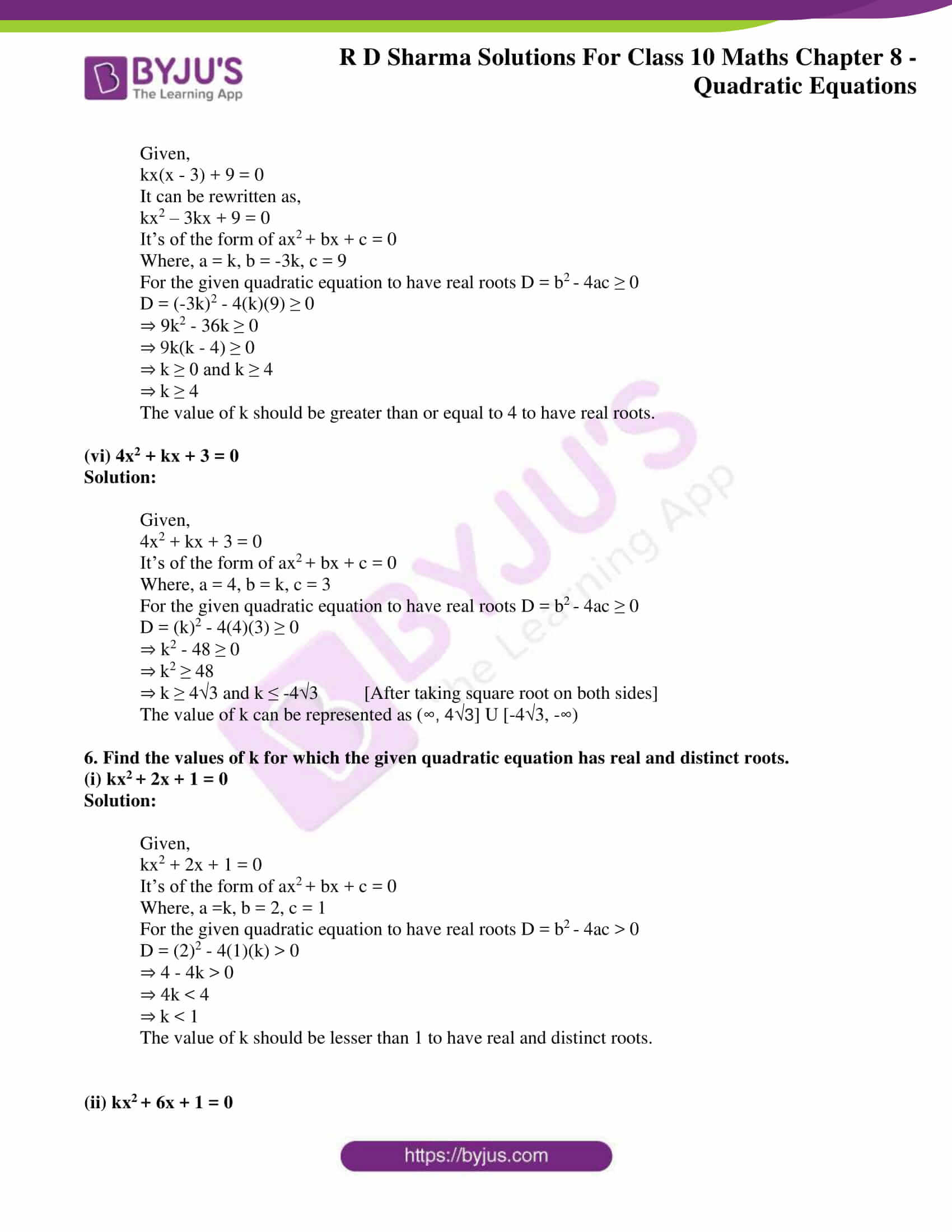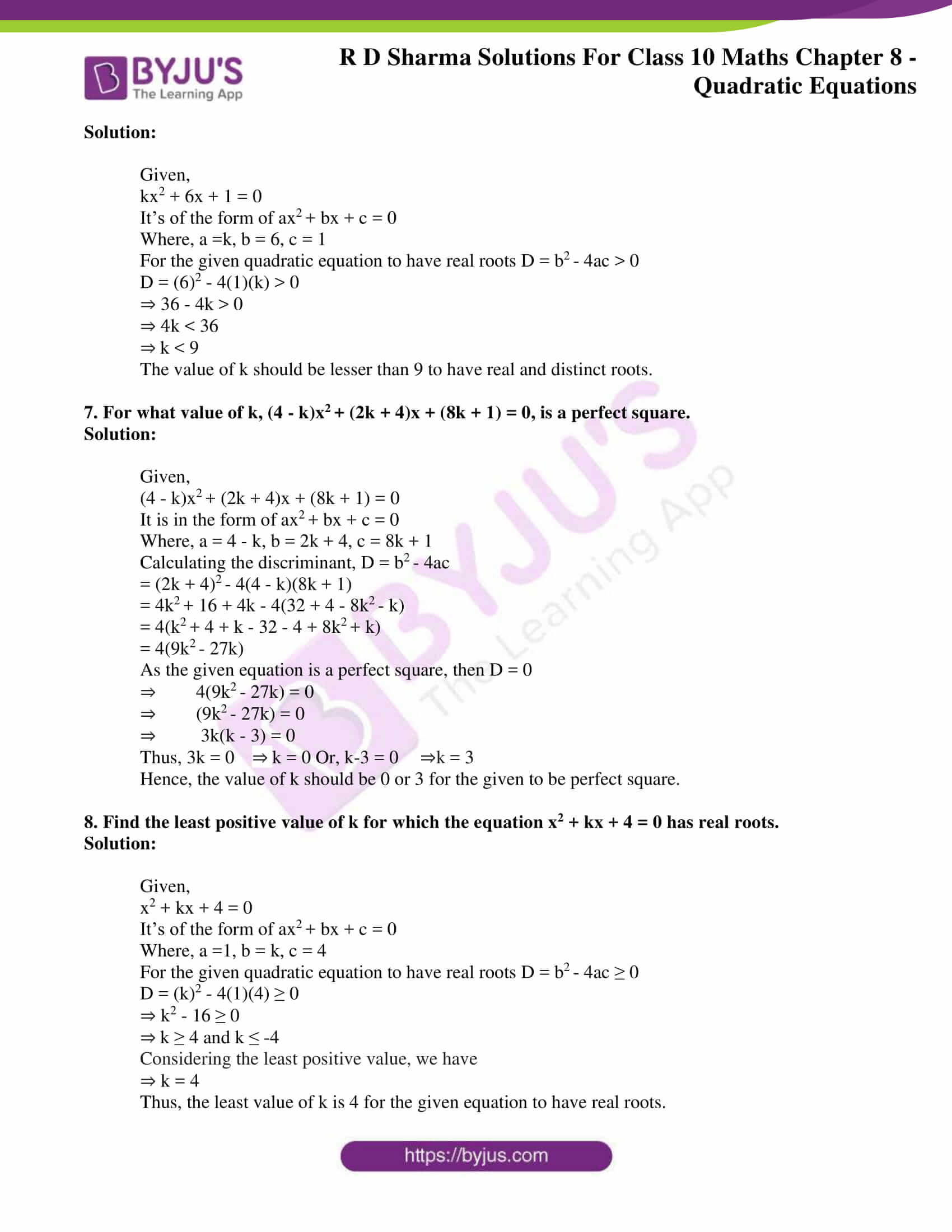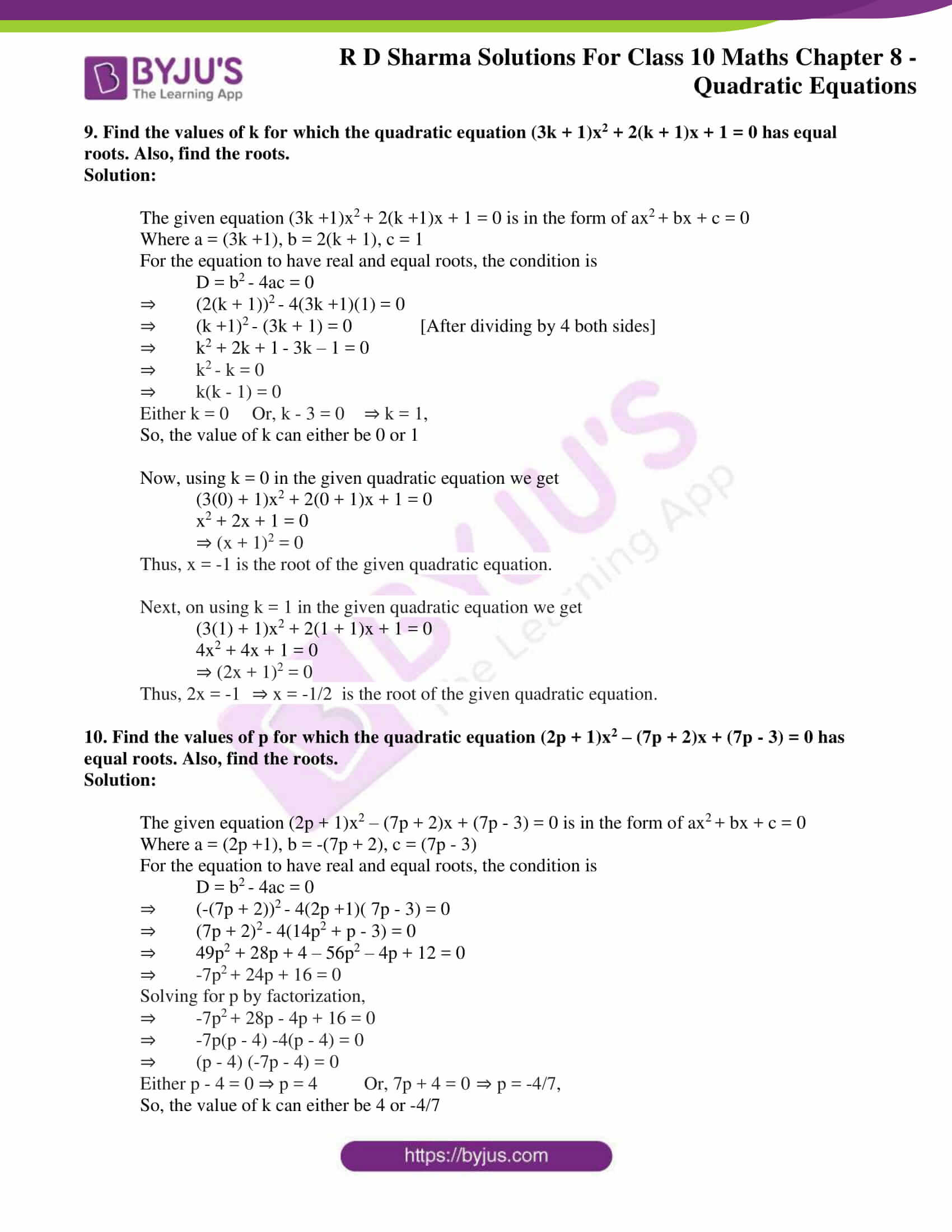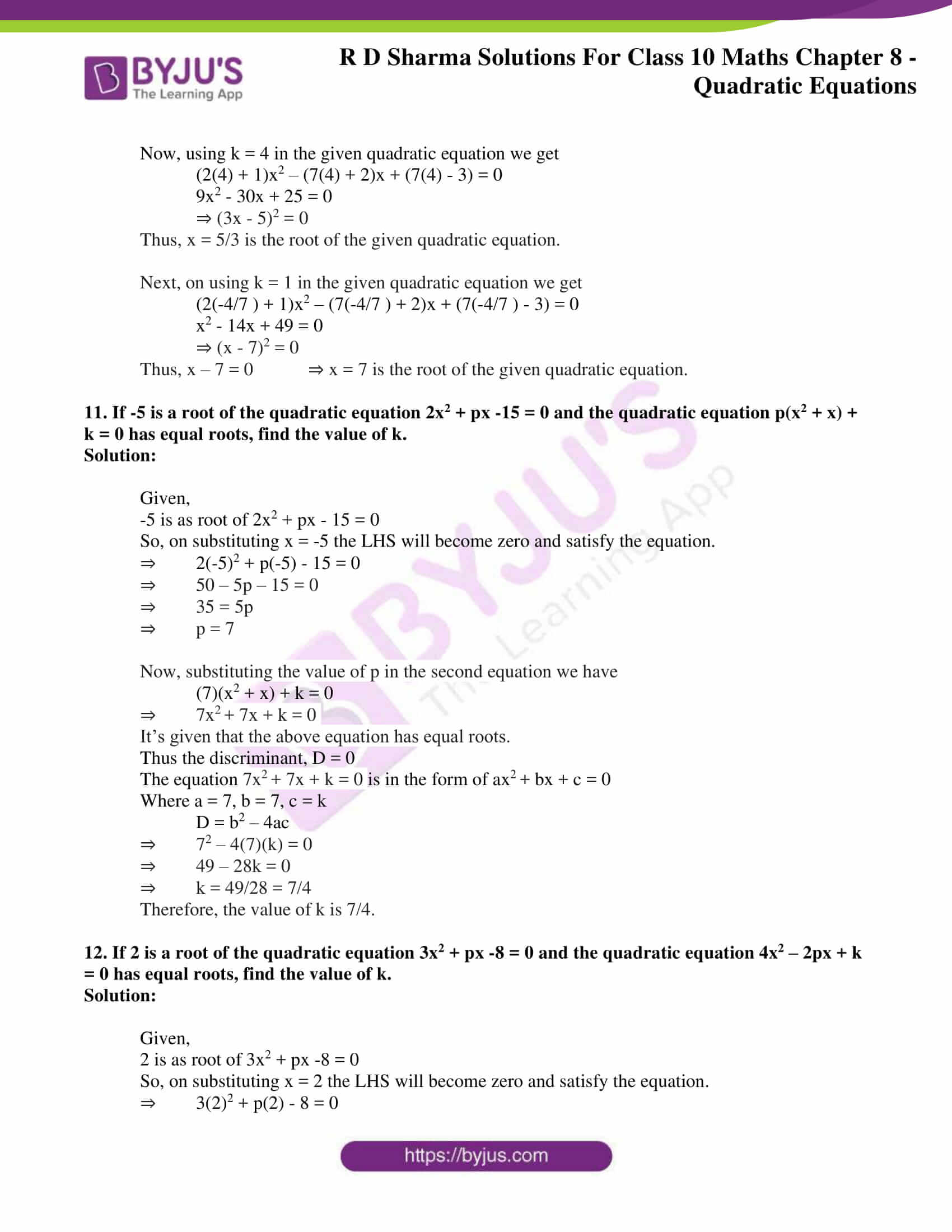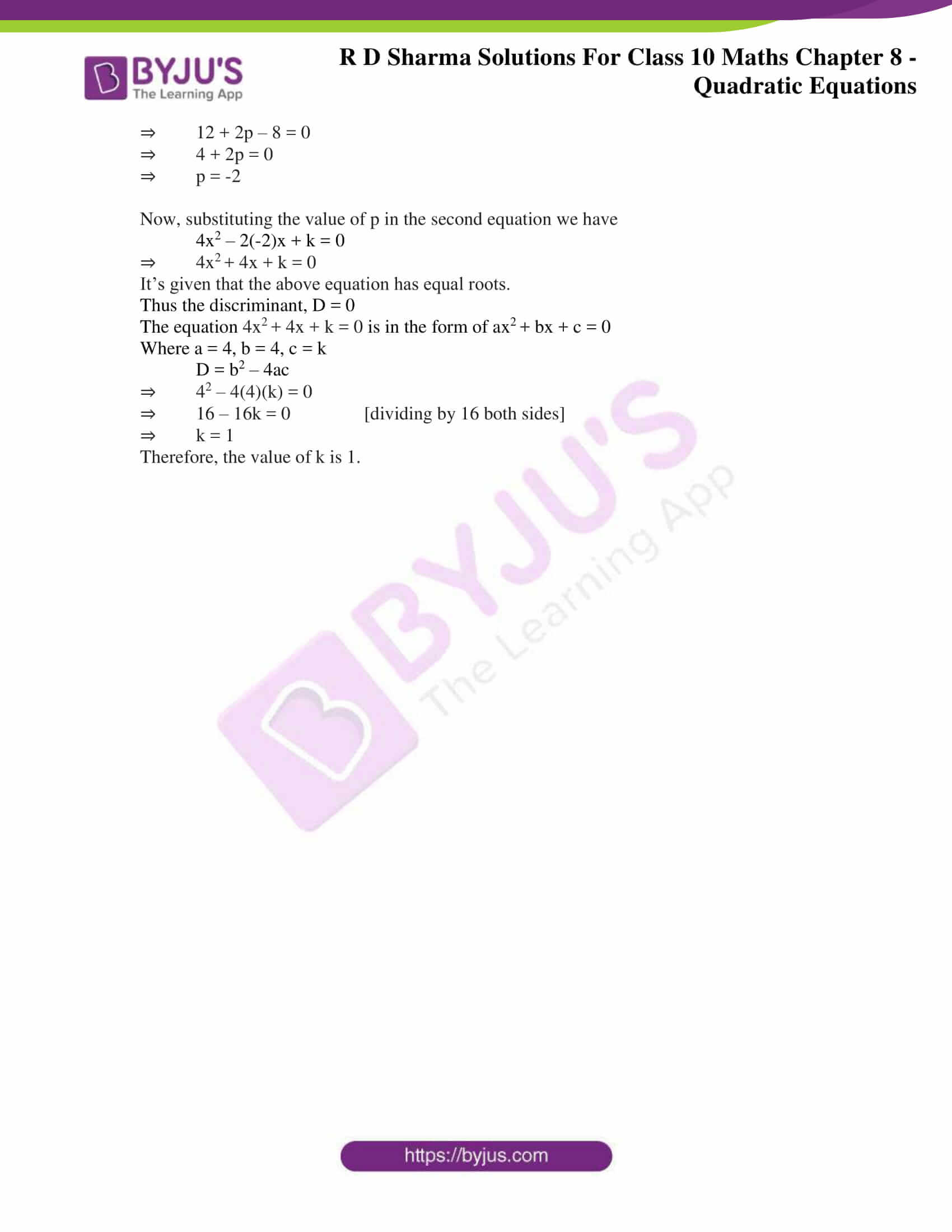### Access RD Sharma Solutions for Class 10 Maths Chapter 8 Quadratic Equations Exercise 8.6

1. Determine the nature of the roots of the following quadratic equations:

Important Notes:

– A quadratic equation is in the form ax+bx +c =0

– To find the nature of roots, first find determinant “D”

– D = b2 – 4ac

– If D > 0, equation has real and distinct roots

– If D < 0, equation has no real roots

– If D = 0, equation has 1 root

(i) 2x2 -3x + 5 =0

Solution:

Here, a= 2, b= -3, c= 5

D = b2 – 4ac

= (-3)2 -4(2)(5)

= 9 – 40

= -31<0

It’s seen that D<0 and hence, the given equation does not have any real roots.

(ii) 2x2 -6x + 3=0

Solution:

Here, a= 2, b= -6, c= 3

D = (-6)2 -4(2)(3)

= 36 – 24

= 12>0

It’s seen that D>0 and hence, the given equation have real and distinct roots.

(iii) (3/5)x2 – (2/3) + 1 = 0

Solution:

Here, a= 3/5, b= -2/3, c= 1

D = (-2/3)2 -4(3/5)(1)

= 4/9 – 12/5

= -88/45<0

It’s seen that D<0 and hence, the given equation does not have any real roots.

(iv) 3x2 – 4√3x + 4 = 0

Solution:

Here, a= 3, b= – 43, c= 4

D = (- 43)2 -4(3)(4)

= 48 – 48

= 0

It’s seen that D = 0 and hence, the given equation has only 1 real and equal root.

(v) 3x2 – 2√6x + 2 = 0

Solution:

Here, a= 3, b= – 26, c= 2

D = (- 26)2 – 4(3)(2)

= 24 – 24

= 0

It’s seen that D = 0 and hence, the given equation has only 1 real and equal root.

2. Find the values of k for which the roots are real and equal in each of the following equations:

(i) kx+ 4x + 1 = 0

Solution:

The given equation kx+ 4x + 1 = 0 is in the form of ax+ bx + c = 0

Where a = k, b = 4, c = 1

For the equation to have real and equal roots, the condition is

D = b– 4ac = 0

⇒ 4– 4(k)(1) = 0

⇒ 16 – 4k = 0

⇒ k = 4

The value of k is 4.

(ii) kx2 – 2√5x + 4 = 0

Solution:

The given equation kx– 2√5x + 4 = 0 is in the form of ax+ bx + c = 0

Where a = k, b = – 2√5, c = 4

For the equation to have real and equal roots, the condition is

D = b– 4ac = 0

⇒ (- 2√5)– 4(k)(4) = 0

⇒ 20 –16k = 0

⇒ k = 5/4

The value of k is 5/4.

(iii) 3x– 5x + 2k = 0

Solution:

The given equation 3x– 5x + 2k = 0 is in the form of ax+ bx + c = 0

Where a = 3, b = -5, c = 2k

For the equation to have real and equal roots, the condition is

D = b– 4ac = 0

⇒ (-5)– 4(3)(2k) = 0

⇒ 25 – 24k = 0

⇒ k = 25/24

The value of k is 25/24.

(iv) 4x+ kx + 9 = 0

Solution:

The given equation 4x+ kx + 9 = 0 is in the form of ax+ bx + c = 0

Where a = 4, b = k, c = 9

For the equation to have real and equal roots, the condition is

D = b– 4ac = 0

⇒ k– 4(4)(9) = 0

⇒ k2 – 144 = 0

⇒ k = ± 12

The value of k is 12 or -12.

(v) 2kx– 40x + 25 = 0

Solution:

The given equation 2kx– 40x + 25 = 0 is in the form of ax+ bx + c = 0

Where a = 2k, b = -40, c = 25

For the equation to have real and equal roots, the condition is

D = b– 4ac = 0

⇒ (-40)– 4(2k)(25) = 0

⇒ 1600 – 200k = 0

⇒ k = 8

The value of k is 8.

(vi) 9x– 24x + k = 0

Solution:

The given equation 9x– 24x + k = 0 is in the form of ax+ bx + c = 0

Where a = 9, b = -24, c = k

For the equation to have real and equal roots, the condition is

D = b– 4ac = 0

⇒ (-24)– 4(9)(k) = 0

⇒ 576 – 36k = 0

⇒ k = 16

The value of k is 16.

(vii) 4x2– 3kx + 1 = 0

Solution:

The given equation 4x– 3kx + 1 = 0 is in the form of ax+ bx + c = 0

Where a = 4, b = -3k, c = 1

For the equation to have real and equal roots, the condition is

D = b– 4ac = 0

⇒ (-3k)– 4(4)(1) = 0

⇒ 9k2 – 16 = 0

⇒ k = ± 4/3

The value of k is ± 4/3.

(viii) x– 2(5 + 2k)x + 3(7 + 10k) = 0

Solution:

The given equation x– 2(5 + 2k)x + 3(7 + 10k) = 0 is in the form of ax+ bx + c = 0

Where a = 1, b = -2(5 + 2k), c = 3(7 + 10k)

For the equation to have real and equal roots, the condition is

D = b– 4ac = 0

⇒ (-2(5 + 2k))– 4(1)( 3(7 + 10k)) = 0

⇒ 4(5 + 2k)– 12(7 + 10k) = 0

⇒ 25 + 4k+ 20k – 21 – 30k = 0

⇒ 4k– 10k + 4 = 0

⇒ 2k– 5k + 2 = 0 [dividing by 2]

Now, solving for k by factorization we have

⇒ 2k– 4k – k + 2 = 0

⇒ 2k(k – 2) – 1(k – 2) = 0

⇒ (k – 2)(2k – 1) = 0,

k = 2 and k = 1/2,

So, the value of k can either be 2 or 1/2

(ix) (3k +1)x2+ 2(k +1)x + k = 0

Solution:

The given equation (3k +1)x+ 2(k +1)x + k = 0 is in the form of ax+ bx + c = 0

Where a = (3k +1), b = 2(k + 1), c = k

For the equation to have real and equal roots, the condition is

D = b– 4ac = 0

⇒ (2(k + 1))– 4(3k +1)(k) = 0

⇒ 4(k +1)– 4(3k2 + k) = 0

⇒ (k + 1)– k(3k + 1) = 0

⇒ 2k– k – 1 = 0

Now, solving for k by factorization we have

⇒ 2k– 2k + k – 1 = 0

⇒ 2k(k – 1) + 1(k – 1) = 0

⇒ (k – 1)(2k + 1) = 0,

k = 1 and k = -1/2,

So, the value of k can either be 1 or -1/2

(x) kx+ kx + 1 = – 4x– x

Solution:

The given equation kx+ kx + 1 = -4x2 – x

This can be rewritten as,

(k + 4)x2 + (k + 1)x + 1 = 0

Now, this in the form of ax+ bx + c = 0

Where a = (k +4), b = (k + 1), c = 1

For the equation to have real and equal roots, the condition is

D = b– 4ac = 0

⇒ (k + 1)– 4(k +4)(1) = 0

⇒ (k +1)– 4k – 16 = 0

⇒ k2 + 2k + 1 – 4k – 16 = 0

⇒ k– 2k – 15 = 0

Now, solving for k by factorization we have

⇒ k– 5k + 3k – 15 = 0

⇒ k(k – 5) + 3(k – 5) = 0

⇒ (k + 3)(k – 5) = 0,

k = -3 and k = 5,

So, the value of k can either be -3 or 5.

(xi) (k + 1)x+ 2(k + 3)x + (k + 8) = 0

Solution:

The given equation (k +1)x+ 2(k +3)x + (k +8) = 0 is in the form of ax+ bx + c = 0

Where a = (k +1), b = 2(k + 3), c = (k + 8)

For the equation to have real and equal roots, the condition is

D = b– 4ac = 0

⇒ (2(k + 3))– 4(k +1)(k + 8) = 0

⇒ 4(k +3)– 4(k2 + 9k + 8) = 0

⇒ (k + 3)– (k2 + 9k + 8) = 0

⇒ k+6k + 9 – k2 – 9k – 8 = 0

⇒ -3k + 1 = 0

⇒ k = 1/3

So, the value of k is 1/3.

(xii) x– 2kx + 7k – 12 = 0

Solution:

The given equation x– 2kx + 7k – 12 = 0 is in the form of ax+ bx + c = 0

Where a = 1, b = -2k, c = 7k – 12

For the equation to have real and equal roots, the condition is

D = b– 4ac = 0

⇒ (-2k)– 4(1)(7k – 12) = 0

⇒ 4k– 4(7k – 12) = 0

⇒ k2 – 7k + 12 = 0

Now, solving for k by factorization we have

⇒ k– 4k – 3k + 12 = 0

⇒ (k – 4)(k – 3) = 0,

k = 4 and k = 3,

So, the value of k can either be 4 or 3.

(xiii) (k + 1)x– 2(3k + 1)x + 8k + 1 = 0

Solution:

The given equation (k +1)x– 2(3k +1)x + 8k + 1 = 0 is in the form of ax+ bx + c = 0

Where a = (k +1), b = -2(3k + 1), c = 8k + 1

For the equation to have real and equal roots, the condition is

D = b– 4ac = 0

⇒ (-2(3k + 1))– 4(k +1)(8k + 1) = 0

⇒ 4(3k +1)– 4(k + 1)(8k + 1) = 0

⇒ (3k + 1)– (k + 1)(8k + 1) = 0

⇒ 9k+ 6k + 1 – (8k+ 9k + 1) = 0

⇒ 9k+ 6k + 1 – 8k– 9k – 1 = 0

⇒ k– 3k = 0

⇒ k(k – 3) = 0

Either k = 0 Or, k – 3 = 0 ⇒ k = 3,

So, the value of k can either be 0 or 3

(xiv) 5x– 4x + 2 + k(4x– 2x + 1) = 0

Solution:

The given equation 5x– 4x + 2 + k(4x– 2x + 1) = 0

This can be rewritten as,

x2(5 + 4k) – x(4 + 2k) + 2 – k = 0

Now, this in the form of ax+ bx + c = 0

Where a = (4k +5), b = -(2k + 4), c = 2 – k

For the equation to have real and equal roots, the condition is

D = b– 4ac = 0

⇒ (-(2k + 4))– 4(4k +5)(2 – k) = 0

⇒ (2k +4)– 4(4k +5)(2 – k) = 0

⇒ 16 + 4k+ 16k – 4(10 – 5k + 8k – 4k2) = 0

⇒ 16 + 4k+ 16k – 40 + 20k – 32k + 16k2 = 0

⇒ 20k+ 4k – 24 = 0

⇒ 5k2 + k – 6 = 0

Now, solving for k by factorization we have

⇒ 5k+ 6k – 5k – 6 = 0

⇒ 5k(k – 1) + 6(k – 1) = 0

⇒ (k – 1)(5k + 6) = 0,

k = 1 and k = -6/5,

So, the value of k can either be 1 or -6/5.

(xv) (4 – k)x+ (2k + 4)x + (8k + 1) = 0

Solution:

The given equation (4 – k)x+ (2k + 4)x + (8k + 1) = 0 is in the form of ax+ bx + c = 0

Where a = (4 – k), b = (2k + 4), c = (8k + 1)

For the equation to have real and equal roots, the condition is

D = b– 4ac = 0

⇒ (2k + 4)– 4(4 – k)(8k + 1) = 0

⇒ 4k+ 16k + 16 – 4(-8k+ 32k + 4 – k) = 0

⇒ 4k+ 16k + 16 + 32k– 124k – 16 = 0

⇒ 36k– 108k = 0

Taking common,

⇒ 9k(k -3 ) = 0

Now, either 9k = 0 ⇒ k = 0 or k – 3 = 0 ⇒ k = 3,

So, the value of k can either be 0 or 3.

(xvi) (2k + 1)x+ 2(k + 3)x + (k +5) = 0

Solution:

The given equation (2k +1)x+ 2(k +3)x + (k + 5) = 0 is in the form of ax+ bx + c = 0

Where a = (2k +1), b = 2(k + 3), c = (k + 5)

For the equation to have real and equal roots, the condition is

D = b– 4ac = 0

⇒ (2(k + 3))– 4(2k +1)(k + 5) = 0

⇒ 4(k +3)– 4(2k2 + 11k + 5) = 0

⇒ (k + 3)– (2k2 + 11k + 5) = 0 [dividing by 4 both sides]

⇒ k+ 5k – 4 = 0

Now, solving for k by completing the square we have

⇒ k+ 2 x (5/2) x k + (5/2)2 = 4 + (5/2)2

⇒ (k + 5/2)2 = 4 + 25/4 = 41/4

⇒ k + (5/2) = ± 41/2

⇒ k = (41 – 5)/2 or –(41 + 5)/2

So, the value of k can either be (41 – 5)/2 or –(41 + 5)/2

(xvii) 4x– 2(k + 1)x + (k + 4) = 0

Solution:

The given equation 4x– 2(k +1)x + (k + 4)= 0 is in the form of ax+ bx + c = 0

Where a = 4, b = -2(k + 1), c = (k + 4)

For the equation to have real and equal roots, the condition is

D = b– 4ac = 0

⇒ (-2(k + 1))– 4(4)(k + 4) = 0

⇒ 4(k +1)– 16(k + 4) = 0

⇒ (k + 1)– 4(k + 4) = 0

⇒ k– 2k – 15 = 0

Now, solving for k by factorization we have

⇒ k– 5k + 3k – 15 = 0

⇒ k(k – 5) + 3(k – 5) = 0

⇒ (k – 5)(k + 3) = 0,

k = 5 and k = -3,

So, the value of k can either be 5 or -3.

3. In the following, determine the set of values of k for which the given quadratic equation has real roots:

(i) 2x+ 3x + k = 0

Solution:

Given,

2x+ 3x + k = 0

It’s of the form of ax+ bx + c = 0

Where, a = 2, b = 3, c = k

For the given quadratic equation to have real roots D = b2– 4ac ≥ 0

D = 9 – 4(2)(k) ≥ 0

⇒ 9 – 8k ≥ 0

⇒ k ≤ 9/8

The value of k should not exceed 9/8 to have real roots.

(ii) 2x+ x + k = 0

Solution:

Given,

2x+ x + k = 0

It’s of the form of ax+ bx + c = 0

Where, a = 2, b = 1, c = k

For the given quadratic equation to have real roots D = b2 – 4ac ≥ 0

D = 12 – 4(2)(k) ≥ 0

⇒ 1 – 8k ≥ 0

⇒ k ≤ 1/8

The value of k should not exceed 1/8 to have real roots.

(iii) 2x– 5x – k = 0

Solution:

Given,

2x– 5x – k = 0

It’s of the form of ax+ bx + c = 0

Where, a = 2, b = -5, c = -k

For the given quadratic equation to have real roots D = b2 – 4ac ≥ 0

D = (-5)2 – 4(2)(-k) ≥ 0

⇒ 25 + 8k ≥ 0

⇒ k ≥ -25/8

The value of k should be lesser than -25/8 to have real roots.

(iv) kx+ 6x + 1 = 0

Solution:

Given,

kx+ 6x + 1 = 0

It’s of the form of ax+ bx + c = 0

Where, a = k, b = 6, c = 1

For the given quadratic equation to have real roots D = b2 – 4ac ≥ 0

D = 62 – 4(k)(1) ≥ 0

⇒ 36 – 4k ≥ 0

The given equation will have real roots if,

36 – 4k ≥ 0

36 ≥ 4k

36/4 ≥ k

9 ≥ k

⇒ so, k ≤ 9

The value of k should not exceed 9 to have real roots.

(v) 3x+ 2x + k = 0

Solution:

Given,

3x+ 2x + k = 0

It’s of the form of ax+ bx + c = 0

Where, a =3, b = 2, c = k

For the given quadratic equation to have real roots D = b2 – 4ac ≥ 0

D = (2)2 – 4(3)(k) ≥ 0

⇒ 4 – 12k ≥ 0

⇒ 4 ≥ 12k

⇒ k ≤ 1/3

The value of k should not exceed 1/3 to have real roots.

4. Find the values of k for which the following equations have real and equal roots

(i) x2 – 2(k + 1)x + k2 = 0

Solution:

Given,

x2 – 2(k + 1)x + k2 = 0

It’s of the form of ax+ bx + c = 0

Where, a =1, b = -2(k + 1), c = k2

For the given quadratic equation to have real roots D = b2 – 4ac = 0

D = (-2(k + 1))2 – 4(1)(k2) = 0

⇒ 4k2 + 8k + 4 – 4k2 = 0

⇒ 8k + 4 = 0

⇒ k = -4/8

⇒ k = -1/2

The value of k should -1/2 to have real and equal roots.

(ii)k2x2 – 2 (2k – 1)x + 4 = 0

Solution:

Given,

k2x2 – 2 (2k – 1)x + 4 = 0

It’s of the form of ax+ bx + c = 0

Where, a = k2, b = –2 (2k – 1), c = 4

For the given quadratic equation to have real roots D = b2 – 4ac = 0

D = (-2(2k – 1))2 – 4(4)(k2) = 0

⇒ 4k2 – 4k + 1 – 4k2 = 0 [dividing by 4 both sides]

⇒ -4k + 1 = 0

⇒ k = 1/4

The value of k should 1/4 to have real and equal roots.

(iii) (k + 1)x2 – 2(k – 1)x + 1 = 0

Solution:

Given,

(k + 1)x2 – 2(k – 1)x + 1 = 0

It’s of the form of ax+ bx + c = 0

Where, a = (k + 1), b = -2(k – 1), c = 1

For the given quadratic equation to have real roots D = b2 – 4ac = 0

D = (-2(k – 1))2 – 4(1)(k + 1) = 0

⇒ 4k2 – 2k + 1 – k – 1 = 0 [dividing by 4 both sides]

⇒ k2 – 3k = 0

⇒ k(k – 3) = 0

⇒ k = 0 or k = 3

The value of k can be 0 or 3 to have real and equal roots.

5. Find the values of k for which the following equations have real roots

(i) 2x2 + kx + 3 = 0

Solution:

Given,

2x+ kx + 3 = 0

It’s of the form of ax+ bx + c = 0

Where, a = 2, b = k, c = 3

For the given quadratic equation to have real roots D = b2 – 4ac ≥ 0

D = (k)2 – 4(3)(2) ≥ 0

⇒ k2 – 24 ≥ 0

⇒ k2 ≥ 24

⇒ k ≥ 26 and k ≤ -26 [After taking square root on both sides]

The value of k can be represented as (∞, 26] U [-26, -∞)

(ii) kx(x – 2) + 6 = 0

Solution:

Given,

kx(x – 2) + 6 = 0

It can be rewritten as,

kx2 – 2kx + 6 = 0

It’s of the form of ax+ bx + c = 0

Where, a =k, b = -2k, c = 6

For the given quadratic equation to have real roots D = b2 – 4ac ≥ 0

D = (-2k)2 – 4(k)(6) ≥ 0

⇒ 4k2 – 24k ≥ 0

⇒ 4k(k – 6) ≥ 0

⇒ k ≥ 0 and k ≥ 6

⇒ k ≥ 6

The value of k should be greater than or equal to 6 to have real roots.

(iii) x2 – 4kx + k = 0

Solution:

Given,

x2 – 4kx + k = 0

It’s of the form of ax+ bx + c = 0

Where, a =1, b = -4k, c = k

For the given quadratic equation to have real roots D = b2 – 4ac ≥ 0

D = (-4k)2 – 4(1)(k) ≥ 0

⇒ 16k2 – 4k ≥ 0

⇒ 4k(4k – 1) ≥ 0

⇒ k ≥ 0 and k ≥ 1/4

⇒ k ≥ 1/4

The value of k should be greater than or equal to 1/4 to have real roots.

(iv) kx(x – 2√5) + 10 = 0

Solution:

Given,

kx(x – 2√5) + 10 = 0

It can be rewritten as,

kx2 – 2√5kx + 10 = 0

It’s of the form of ax+ bx + c = 0

Where, a =k, b = -2√5k, c = 10

For the given quadratic equation to have real roots D = b2 – 4ac ≥ 0

D = (-2√5k)2 – 4(k)(10) ≥ 0

⇒ 20k2 – 40k ≥ 0

⇒ 20k(k – 2) ≥ 0

⇒ k ≥ 0 and k ≥ 2

⇒ k ≥ 2

The value of k should be greater than or equal to 2 to have real roots.

(v) kx(x – 3) + 9 = 0

Solution:

Given,

kx(x – 3) + 9 = 0

It can be rewritten as,

kx2 – 3kx + 9 = 0

It’s of the form of ax+ bx + c = 0

Where, a = k, b = -3k, c = 9

For the given quadratic equation to have real roots D = b2 – 4ac ≥ 0

D = (-3k)2 – 4(k)(9) ≥ 0

⇒ 9k2 – 36k ≥ 0

⇒ 9k(k – 4) ≥ 0

⇒ k ≥ 0 and k ≥ 4

⇒ k ≥ 4

The value of k should be greater than or equal to 4 to have real roots.

(vi) 4x2 + kx + 3 = 0

Solution:

Given,

4x2 + kx + 3 = 0

It’s of the form of ax+ bx + c = 0

Where, a = 4, b = k, c = 3

For the given quadratic equation to have real roots D = b2 – 4ac ≥ 0

D = (k)2 – 4(4)(3) ≥ 0

⇒ k2 – 48 ≥ 0

⇒ k2 ≥ 48

⇒ k ≥ 43 and k ≤ -43 [After taking square root on both sides]

The value of k can be represented as (∞, 43] U [-43, -∞)

6. Find the values of k for which the given quadratic equation has real and distinct roots.

(i) kx+ 2x + 1 = 0

Solution:

Given,

kx2 + 2x + 1 = 0

It’s of the form of ax+ bx + c = 0

Where, a =k, b = 2, c = 1

For the given quadratic equation to have real roots D = b2 – 4ac > 0

D = (2)2 – 4(1)(k) > 0

⇒ 4 – 4k > 0

⇒ 4k < 4

⇒ k < 1

The value of k should be lesser than 1 to have real and distinct roots.

(ii) kx+ 6x + 1 = 0

Solution:

Given,

kx2 + 6x + 1 = 0

It’s of the form of ax+ bx + c = 0

Where, a =k, b = 6, c = 1

For the given quadratic equation to have real roots D = b2 – 4ac > 0

D = (6)2 – 4(1)(k) > 0

⇒ 36 – 4k > 0

⇒ 4k < 36

⇒ k < 9

The value of k should be lesser than 9 to have real and distinct roots.

7. For what value of k, (4 – k)x+ (2k + 4)x + (8k + 1) = 0, is a perfect square.

Solution:

Given,

(4 – k)x+ (2k + 4)x + (8k + 1) = 0

It is in the form of ax+ bx + c = 0

Where, a = 4 – k, b = 2k + 4, c = 8k + 1

Calculating the discriminant, D = b– 4ac

= (2k + 4)– 4(4 – k)(8k + 1)

= 4k+ 16 + 4k – 4(32 + 4 – 8k– k)

= 4(k+ 4 + k – 32 – 4 + 8k+ k)

= 4(9k– 27k)

As the given equation is a perfect square, then D = 0

⇒ 4(9k– 27k) = 0

⇒ (9k– 27k) = 0

⇒ 3k(k – 3) = 0

Thus, 3k = 0 ⇒ k = 0 Or, k-3 = 0 ⇒k = 3

Hence, the value of k should be 0 or 3 for the given to be perfect square.

8. Find the least positive value of k for which the equation x2 + kx + 4 = 0 has real roots.

Solution:

Given,

x2 + kx + 4 = 0

It’s of the form of ax+ bx + c = 0

Where, a =1, b = k, c = 4

For the given quadratic equation to have real roots D = b2 – 4ac ≥ 0

D = (k)2 – 4(1)(4) ≥ 0

⇒ k2 – 16 ≥ 0

⇒ k ≥ 4 and k ≤ -4

Considering the least positive value, we have

⇒ k = 4

Thus, the least value of k is 4 for the given equation to have real roots.

9. Find the values of k for which the quadratic equation (3k + 1)x2 + 2(k + 1)x + 1 = 0 has equal roots. Also, find the roots.

Solution:

The given equation (3k +1)x+ 2(k +1)x + 1 = 0 is in the form of ax+ bx + c = 0

Where a = (3k +1), b = 2(k + 1), c = 1

For the equation to have real and equal roots, the condition is

D = b– 4ac = 0

⇒ (2(k + 1))– 4(3k +1)(1) = 0

⇒ (k +1)– (3k + 1) = 0 [After dividing by 4 both sides]

⇒ k2 + 2k + 1 – 3k – 1 = 0

⇒ k– k = 0

⇒ k(k – 1) = 0

Either k = 0 Or, k – 3 = 0 ⇒ k = 1,

So, the value of k can either be 0 or 1

Now, using k = 0 in the given quadratic equation we get

(3(0) + 1)x2 + 2(0 + 1)x + 1 = 0

x2 + 2x + 1 = 0

⇒ (x + 1)2 = 0

Thus, x = -1 is the root of the given quadratic equation.

Next, on using k = 1 in the given quadratic equation we get

(3(1) + 1)x2 + 2(1 + 1)x + 1 = 0

4x2 + 4x + 1 = 0

⇒ (2x + 1)2 = 0

Thus, 2x = -1 ⇒ x = -1/2 is the root of the given quadratic equation.

10. Find the values of p for which the quadratic equation (2p + 1)x2 – (7p + 2)x + (7p – 3) = 0 has equal roots. Also, find the roots.

Solution:

The given equation (2p + 1)x2 – (7p + 2)x + (7p – 3) = 0 is in the form of ax+ bx + c = 0

Where a = (2p +1), b = -(7p + 2), c = (7p – 3)

For the equation to have real and equal roots, the condition is

D = b– 4ac = 0

⇒ (-(7p + 2))– 4(2p +1)( 7p – 3) = 0

⇒ (7p + 2)– 4(14p2 + p – 3) = 0

⇒ 49p2 + 28p + 4 – 56p2 – 4p + 12 = 0

⇒ -7p+ 24p + 16 = 0

Solving for p by factorization,

⇒ -7p+ 28p – 4p + 16 = 0

⇒ -7p(p – 4) -4(p – 4) = 0

⇒ (p – 4) (-7p – 4) = 0

Either p – 4 = 0 ⇒ p = 4 Or, 7p + 4 = 0 ⇒ p = -4/7,

So, the value of k can either be 4 or -4/7

Now, using k = 4 in the given quadratic equation we get

(2(4) + 1)x2 – (7(4) + 2)x + (7(4) – 3) = 0

9x2 – 30x + 25 = 0

⇒ (3x – 5)2 = 0

Thus, x = 5/3 is the root of the given quadratic equation.

Next, on using k = 1 in the given quadratic equation we get

(2(-4/7 ) + 1)x2 – (7(-4/7 ) + 2)x + (7(-4/7 ) – 3) = 0

x2 – 14x + 49 = 0

⇒ (x – 7)2 = 0

Thus, x – 7 = 0 ⇒ x = 7 is the root of the given quadratic equation.

11. If -5 is a root of the quadratic equation 2x2 + px -15 = 0 and the quadratic equation p(x2 + x) + k = 0 has equal roots, find the value of k.

Solution:

Given,

-5 is as root of 2x2 + px – 15 = 0

So, on substituting x = -5 the LHS will become zero and satisfy the equation.

⇒ 2(-5)2 + p(-5) – 15 = 0

⇒ 50 – 5p – 15 = 0

⇒ 35 = 5p

⇒ p = 7

Now, substituting the value of p in the second equation we have

(7)(x2 + x) + k = 0

⇒ 7x2 + 7x + k = 0

It’s given that the above equation has equal roots.

Thus the discriminant, D = 0

The equation 7x2 + 7x + k = 0 is in the form of ax+ bx + c = 0

Where a = 7, b = 7, c = k

D = b2 – 4ac

⇒ 72 – 4(7)(k) = 0

⇒ 49 – 28k = 0

⇒ k = 49/28 = 7/4

Therefore, the value of k is 7/4.

12. If 2 is a root of the quadratic equation 3x2 + px -8 = 0 and the quadratic equation 4x2 – 2px + k = 0 has equal roots, find the value of k.

Solution:

Given,

2 is as root of 3x2 + px -8 = 0

So, on substituting x = 2 the LHS will become zero and satisfy the equation.

⇒ 3(2)2 + p(2) – 8 = 0

⇒ 12 + 2p – 8 = 0

⇒ 4 + 2p = 0

⇒ p = -2

Now, substituting the value of p in the second equation we have

4x2 – 2(-2)x + k = 0

⇒ 4x2 + 4x + k = 0

It’s given that the above equation has equal roots.

Thus the discriminant, D = 0

The equation 4x2 + 4x + k = 0 is in the form of ax+ bx + c = 0

Where a = 4, b = 4, c = k

D = b2 – 4ac

⇒ 42 – 4(4)(k) = 0

⇒ 16 – 16k = 0 [dividing by 16 both sides]

⇒ k = 1

Therefore, the value of k is 1.